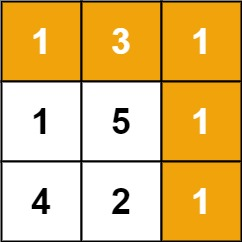Given a `m x n` `grid` filled with non-negative numbers, find a path from top left to bottom right, which minimizes the sum of all numbers along its path.

Note: You can only move either down or right at any point in time.

Example 1:```Input: grid = [[1,3,1],[1,5,1],[4,2,1]]
Output: 7
Explanation: Because the path 1 → 3 → 1 → 1 → 1 minimizes the sum.
```

Example 2:

```Input: grid = [[1,2,3],[4,5,6]]
Output: 12
```

Constraints:

• `m == grid.length`
• `n == grid[i].length`
• `1 <= m, n <= 200`
• `0 <= grid[i][j] <= 100`

Idea:

Dynamic Programing

``````save space: we just use the original grid to calcuate the min path sum
grid[i][j] represent the min path sum of i x j grid
grid[i][j] = get the min of previous grid(top/left) + current val``````

Solution:

``````/**
* @param {number[][]} grid
* @return {number}
*/
var minPathSum = function(grid) {
// save space: we just use the original grid to calcuate the min path sum
// grid[i][j] = min path sum of i x j grid
let m = grid.length;
let n = grid.length;

for (let i = 0; i < m; i++) {
for (let j = 0; j < n; j++) {
// grid itself contains the min path sum
if (i === 0 && j === 0) continue;
// first row: grid[i][j] = previous grid(left) + current val
if (i === 0) grid[i][j] += grid[i][j - 1];
// first column: grid[i][j] = previous grid(top) + current val
else if (j === 0) grid[i][j] += grid[i - 1][j];
// grid[i][j] = get the min of previous grid(top/left) + current val
else grid[i][j] += Math.min(grid[i][j - 1], grid[i - 1][j]);
}
}
return grid[m - 1][n - 1];
}
``````